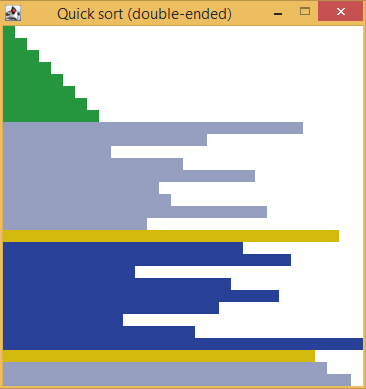#Sorting algorithms demo (Java)This Java desktop GUI program shows an animation of various sorting algorithms in action, such as bubble sort or quicksort. It includes these 19 sorting algorithms (listed from fastest to slowest):

• Quicksort (double-ended) (O(n log n))
• Quicksort (sliding) (O(n log n))
• Heapsort (O(n log n))
• Shell sort (~O(n1.3))
• Comb sort (~O(n2))
• Rotation merge sort (~O(n2))
• Insertion sort (with binary search) (O(n2))
• Insertion sort (O(n2))
• Bubble sort (O(n2))
• Cocktail sort (O(n2))
• Gnome sort (O(n2))
• Pancake sort (O(n2))
• Quasi-pancake sort (O(n2))
• Selection sort (O(n2))
• Cycle sort (O(n2))
• Stooge sort (O(n2.71))
• Stupid sort (O(n3))
• Bozo sort (~O(n3 log n))
• Slowsort (O(nlog n))

On Windows, simply double click the JAR file to run it. Otherwise, type this on a command line: `java -jar nayuki-sort-demo.jar`

This contains source code for the application classes but no unit tests.

## Source code

Browse the complete source code at GitHub:https://github.com/nayuki/Sorting-algorithms-demo

Or download a ZIP of all the files:https://github.com/nayuki/Sorting-algorithms-demo/archive/master.zip

The code package also includes JUnit test cases for every sorting algorithm to ensure that they are implemented correctly.

The source code is open source under the MIT license.

## Videos

Note that the videos run at different speeds, so the length of the video is not a reliable indicator of the sorting algorithm’s speed. Check the number of comparisons and swaps to see how much work was actually performed by the algorithm.